# Images

Images are stored in `ColorBuffer` instances, the image data resides in GPU memory

Images are loaded using the `loadImage` function and drawn using `Drawer.image`.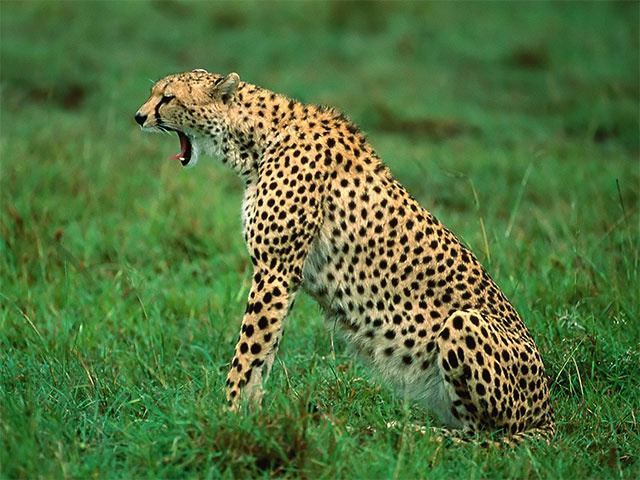``````fun main() = application {
configure {}
program {

extend {
drawer.image(image)
}
}
}
``````

To change the location of the image one can use `Drawer.image` with extra coordinates provided.

``````drawer.image(image, 40.0, 40.0)
``````

Extra `width` and `height` arguments can be provided to draw a scaled version of the image

``````drawer.image(image, 40.0, 40.0, 64.0, 48.0)
``````

## Drawing parts of images

It is possible to draw parts of images by specifying source and target rectangles. The source rectangle describes the area that should be taken from the image and presented in the target rectangle.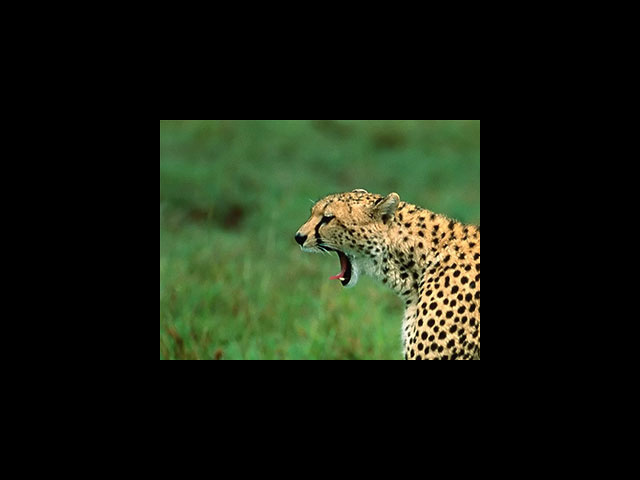``````fun main() = application {
program {

extend {
val source = Rectangle(0.0, 0.0, 320.0, 240.0)
val target = Rectangle(160.0, 120.0, 320.0, 240.0)
drawer.image(image, source, target)
}
}
}
``````

## Drawing many parts of images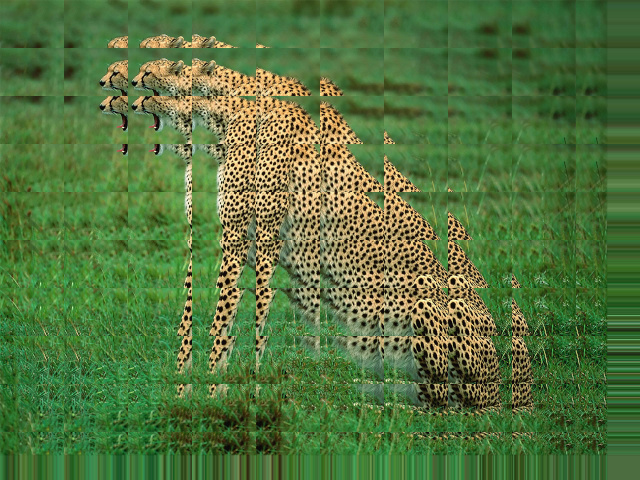``````fun main() = application {
program {

extend {
val areas = (0..10).flatMap { y ->
(0..10).map { x ->
val source = Rectangle(x * (width / 10.0), y * (height / 10.0), width / 5.0, height / 5.0)
val target = Rectangle(x * (width / 10.0), y * (height / 10.0), width / 10.0, height / 10.0)
source to target
}
}
drawer.image(image, areas)
}
}
}
``````

## Changing the appearance of images

A linear color transform can be applied to images by setting `Drawer.drawStyle.colorMatrix` to a `Matrix55` value.

### Tinting

Tinting multiplies the image color with a tint color.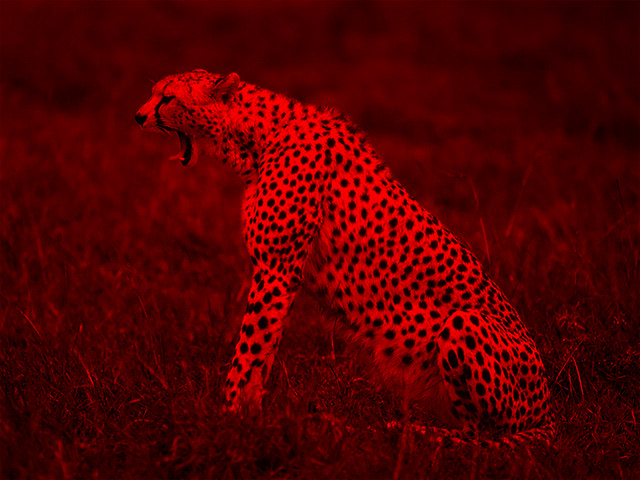``````fun main() = application {
program {

extend {
drawer.drawStyle.colorMatrix = tint(ColorRGBa.RED)
drawer.image(image, 0.0, 0.0)
}
}
}
``````

### Inverting

Drawing an image with inverted colors can be achieved by using the `invert` color matrix.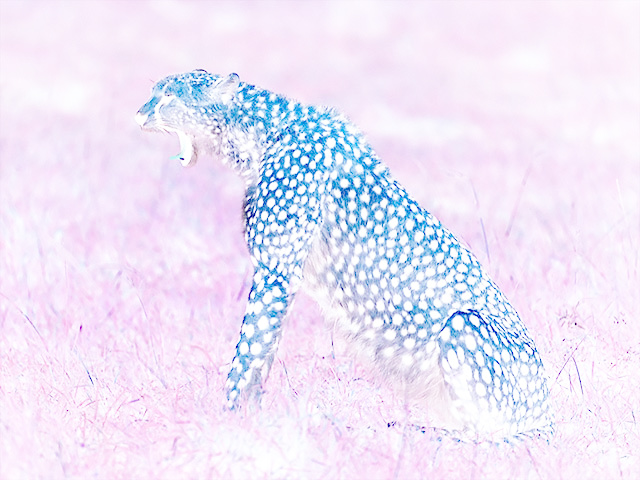``````fun main() = application {
program {

extend {
drawer.drawStyle.colorMatrix = invert
drawer.image(image, 0.0, 0.0)
}
}
}
``````

### Grayscale

Drawing an image in grayscale can be achieved by using the `grayscale` color matrix.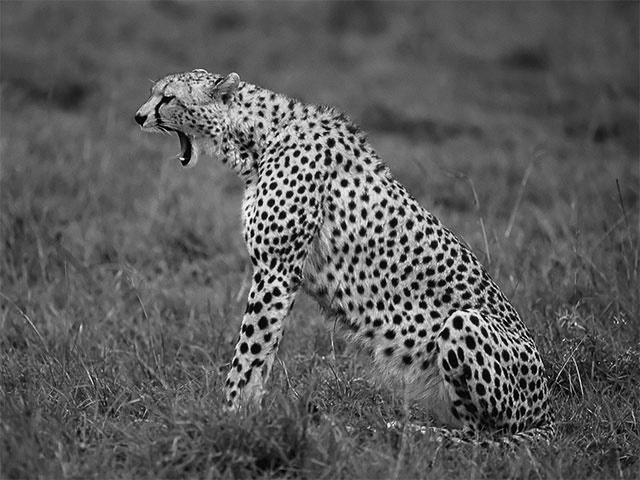``````fun main() = application {
program {

extend {
// -- the factors below determine the RGB mixing factors
drawer.drawStyle.colorMatrix = grayscale(1.0 / 3.0, 1.0 / 3.0, 1.0 / 3.0)
drawer.image(image)
}
}
}
``````

### Concatenating color transforms

Color transforms can be combined using the multiplication operator. This is called transform concatenation. Keep in mind that transform concatenations are read from right to left, and in the following example we first apply the `grayscale` transform and then the `tint` transform.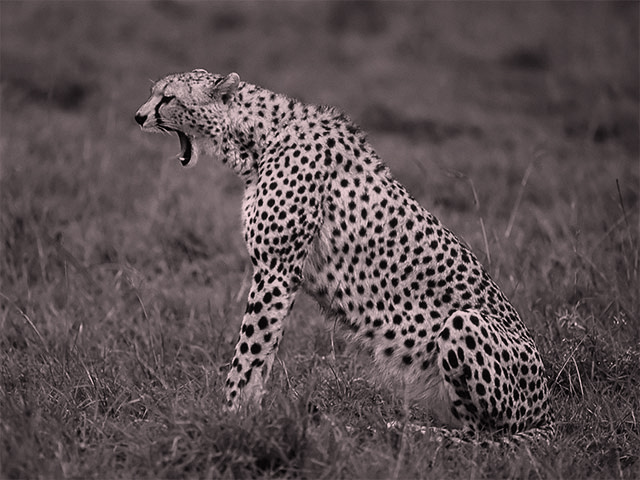``````fun main() = application {
program {